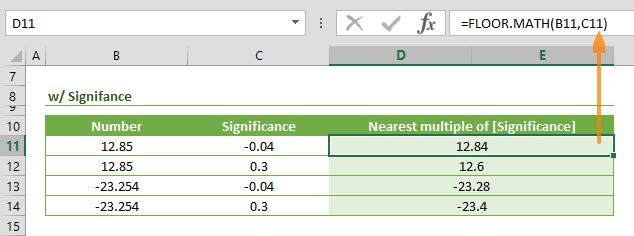The FLOOR MATH (FLOOR.MATH) function is a Math formula that rounds a given number down to the nearest multiple of significance. This function was released in Excel 2013 as a replacement for the FLOOR function. In this guide, we’re going to show you how to use the FLOOR MATH function and also go over some tips and error handling methods.

## Supported versions

• Excel 2013 and newer versions

## FLOOR MATH Function Syntax

FLOOR.MATH(number,[significance],[mode])

## Arguments

 number The number you want to round down. [significance] Optional. The multiple to which you want to round. Default is 1. [mode] Optional. The direction to round negative numbers. Default is 0. Use a negative number to change direction of the rounding for negative numbers.

## Examples

### Rounding down to the nearest integer

If both optional arguments are omitted, the FLOOR MATH function rounds the number argument down to the nearest integer. The direction is away from 0 for negative numbers as well.

=FLOOR.MATH(-23.254) returns -24### Rounding down to the nearest multiple of significance

Enter a [significance] value to round the number down to a specific multiple. You can use decimal values or integers. If you add the [significance] without the [mode] argument, FLOOR MATH rounds negative numbers away from zero by default.

=FLOOR.MATH(-23.254,-0.04) returns -23.28### Rounding down to the nearest multiple of significance by mode

The [mode] argument specifies the rounding direction only for negative numbers. Any value other than zero (0), the default value, reverts the direction. Thus, the FLOOR MATH function will round negative numbers towards zero.

=FLOOR.MATH(-23.254,-0.04,1) returns -23.24

=FLOOR.MATH(-23.254,-0.04,0) returns -23.28## Summary and Tips

• Excel introduced the FLOOR MATH function as the successor of the FLOOR function. The support for FLOOR function continues for the sake of backwards compatibility.
• Use the CEILING.MATH function to round up to the nearest multiple.
• If you need to round to nearest multiple, up or down, use MROUND.
• The [mode] argument doesn't have any effect on positive numbers.